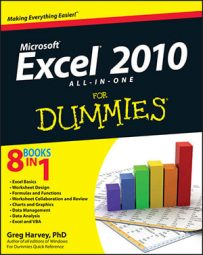##### Excel 2010 All-in-One For DummiesExcel 2010 uses seven logical functions — AND, FALSE, IF, IFERROR, NOT, OR, and TRUE — which appear on the Logical command button's drop-down menu on the Formulas tab of the Ribbon. All the logical functions return either the logical TRUE or logical FALSE when their functions are evaluated.

Here are the names of the logical functions along with their argument syntax:

• AND(logical1,logical2,...) tests whether the logical arguments are TRUE or FALSE. If they are all TRUE, the AND function returns TRUE to the cell. If any are FALSE, the AND function returns FALSE.

• IF(logical_test,value_if_true,value_if_false) tests whether the logical_test expression is TRUE or FALSE. If TRUE, the IF function returns the value_if_true argument. If FALSE, the IF function returns the value_if_false argument.

• IFERROR(value,value_if_error) tests whether the value expression is an error. IFERROR returns value_if_error if the expression is an error, or value of the expression if it is not an error.

• NOT(logical) tests whether the logical argument is TRUE or FALSE. If TRUE, the NOT function returns FALSE. IF FALSE, the NOT function returns TRUE.

• OR(logical1,logical2,...) tests whether the logical arguments are TRUE or FALSE. If any are TRUE, the OR function returns TRUE. If all are FALSE, the OR function returns FALSE.

• FALSE() takes no argument and simply enters logical FALSE in its cell.

• TRUE() takes no argument and simply enters logical TRUE in its cell.

The logical_test and logical arguments that you specify for these logical functions usually employ the comparison operators (=, <, >, <=, >=, and <>), which themselves return logical TRUE or logical FALSE values. For example, suppose that you enter the following formula in your worksheet:

`=AND(B5=D10,C15>=500)`

In this formula, Excel first evaluates the first logical argument to determine whether the contents in cell B5 and D10 are equal to each other. If they are, the first comparison returns TRUE. If they are not equal to each other, this comparison returns FALSE. The program then evaluates the second logical argument to determine whether the content of cell C15 is greater than or equal to 500. If it is, the second comparison returns TRUE. If it is not greater than or equal to 500, this comparison returns FALSE.

After evaluating the comparisons in the two logical arguments, the AND function compares the results: If logical argument 1 and logical argument 2 are both found to be TRUE, then the AND function returns logical TRUE to the cell. If, however, either argument is found to be FALSE, the AND function returns FALSE to the cell.

When you use the IF function, you specify what's called a logical_test argument whose outcome determines whether the value_if_true or value_if_false argument is evaluated and returned to the cell. The logical_test argument normally uses comparison operators, which return either the logical TRUE or logical FALSE value. When the argument returns TRUE, the entry or expression in the value_if_true argument is returned to the cell. When the argument returns FALSE, the entry or expression in the value_if_false argument is returned.

Consider the following formula that uses the IF function to determine whether to charge tax on an item:

`=IF(E5="Yes",D5+D5*7.5%,D5)`

If cell E5 contains Yes, the IF function uses the value_if_true argument that tells Excel to add the price entered in cell D5 to the same value times the tax rate of 7.5%. If, however, cell D5 is blank or contains anything other than the text Yes, then the IF function uses the value_if_false argument, which tells Excel to just return the price in cell D5 without adding any tax to it.

As you can see, the value_if_true and value_if_false arguments of the IF function can contain constants or expressions whose results are returned to the cell that holds the IF formula.

Did this glimpse into Excel formulas and functions leave you longing for more information and insight about Microsoft's popular spreadsheet program? You're free to test drive any of the For Dummies eLearning courses. Pick your course (you may be interested in more from Excel 2010), fill out a quick registration, and then give eLearning a spin with the Try It! button. You'll be right on course for more trusted know how: The full version's also available at Excel 2010.